## Hammer of Math: Does rolling order matter?

An article by    Hammer of Math Warhammer 40k        0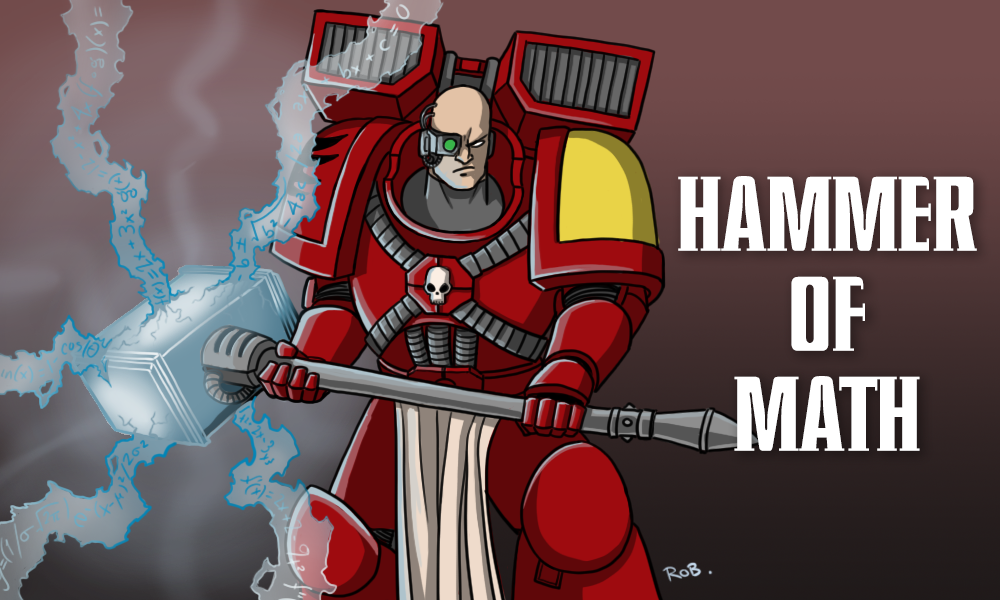This week’s article is a Quick Hit from a reader question. If you have a question that you think would make for a good article, feel free to submit it and I might use it in a future article.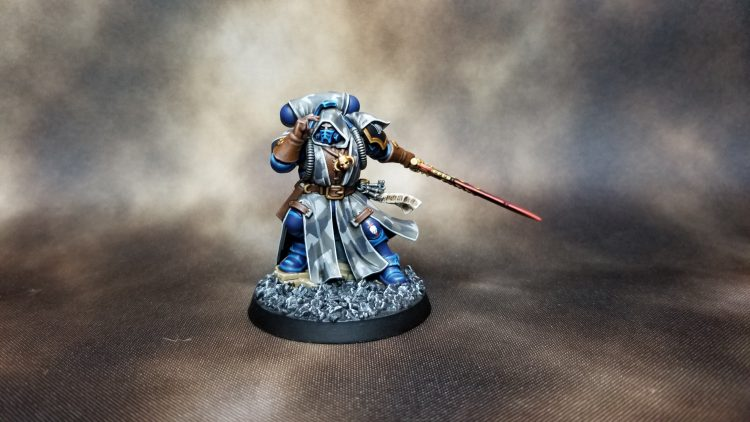Raven Guard Phobos Librarian. Credit: Dan Boyd

I’ve always found it odd that we roll to wound before rolling armour saves – in real life it (I imagine) generally works the other way round. What would be the most unexpected consequence of switching the order in 9th edition?

In 40K the order of operations is somewhat unusual. You roll to see if you hit the target, roll to see if the hit wounds, roll to see if that wounding strike penetrates the armor, then roll to determine how much damage is dealt. Then if the target has an ability to ignore wounds each resulting wound is rolled. Going back over a year ago we know from the first article in this series that the resulting outcome is a product of several probabilities. Assuming there’s no ability to ignore wounds and that all of the probabilities are independent (meaning no roll affects the other, for example a weapon that automatically wounds on a hit of 6), then the equation for the probability of damage is below.

P(Damage) = P(Hit) * P(Wound) * (1 – P(Save))

Because all of these probabilities are independent, and because multiplication is commutative (meaning you can switch the order of operations around with affecting the outcome), this means that the order of operations for the basic Hit -> Wound -> Save progression is irrelevant. Sure enough if we apply these values to a sequence of dice rolls we get that the two overlap. The slight variation in curves for the chart below is due to the fact that the chart was taken from a simulation of 100,000 rolls. If you use an exact solution like the Warhammer Stats Engine then the curves will overlap perfectly.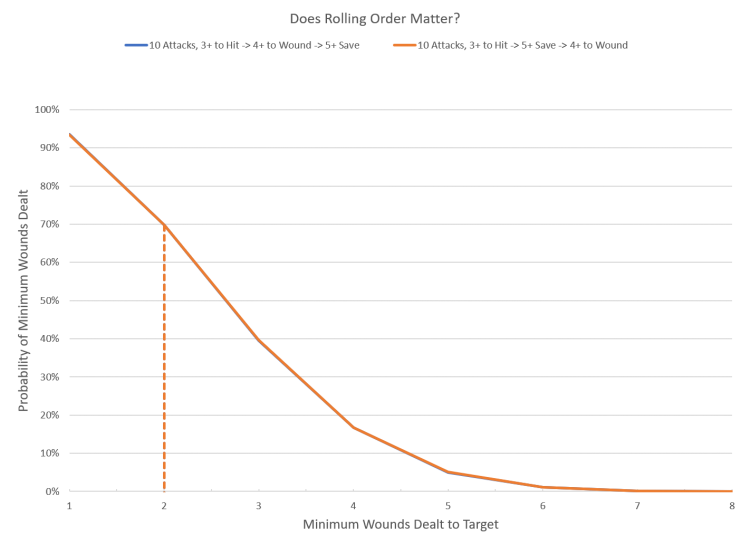The commutative property is also why there’s no difference between re-rolling a 1 on the Hit or Wound roll (assuming nothing extra procs on a particular result). The 1 in 6 improvement is applied equally.

P(Hit, RR1) = P(Hit) + (1/6) * P(Hit) = (7/6) * P(Hit)

P(Damage, RR1 Hit) = 7/6 * P(Hit) * P(Wound) * (1 – P(Save))

P(Wound, RR1) = P(Wound) + (1/6)*P(Wound) = (7/6) * P(Wound)

P(Damage, RR1 Wound) = 7/6 * P(Hit) * P(Wound) * (1 – P(Save))

We see that the two values are equal, and this falls out in the simulation as well. Even though the Hit roll and Wound rolls have different target values, the distribution of wounds is the same. Note that this does not apply when the re-roll is dynamic, meaning that the target number changes based on the circumstances (typically seen as re-rolling failed rolls). In that case the effect is greater when re-rolling lower probabilities; in other words the more chances there are to re-roll a die the more of an impact that the re-roll will have on the overall probability of success. Re-rolling a 3+ improves the overall probability by (1 + 1/3) or 133%, while re-rolling a 4+ improves the overall probability by (1 + 1/2) or 150%.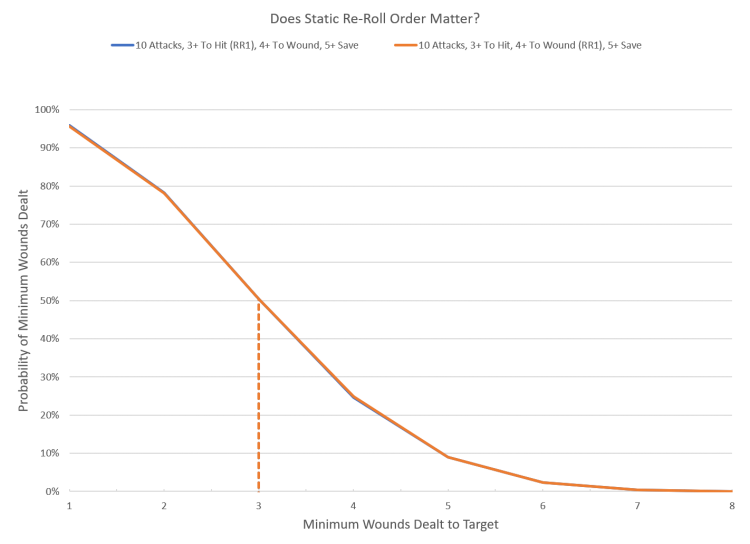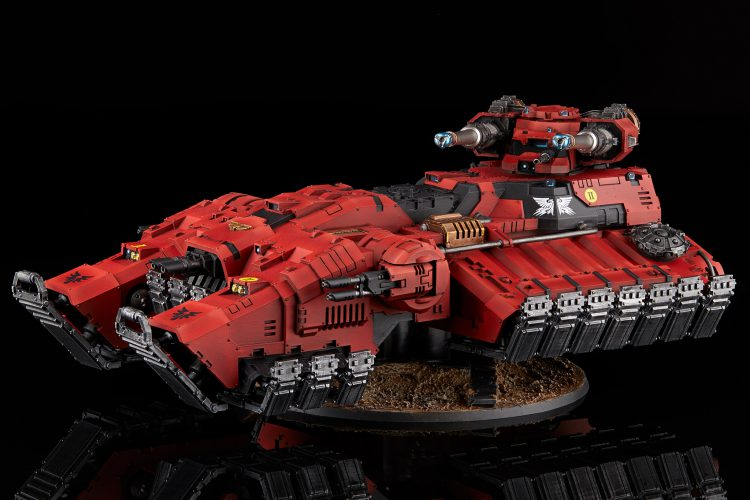Blood Angels Astraeus Grav-Tank. Credit: Jack Hunter

So is there an order of operations that would cause a meaningful shift in the expected outcome? Yes, but it requires three changes:

1. You put the Save roll immediately after the Hit roll.
2. You determine the amount of damage dealt after the save is failed.
3. You roll a Wound roll for each point of damage dealt.

By changing the order of operations in this fashion the average wounds dealt remains the same, but the probability distribution shifts to a smoother curve. This produces the same effect as the various ignore wounds abilities which apply to each individual wound dealt. The end result is that the mean result is the same, but the outcomes are far less ‘swingy’ than they currently are. This is the only way that changing the order of operations of an attack sequence would result in changing the outcome. Whether or not it’s a better choice is a matter of personal preference (I’m a fan, plus it means a ‘wound’ roll finally causes wounds), but it’s an example of how to change the outcome by changing the order.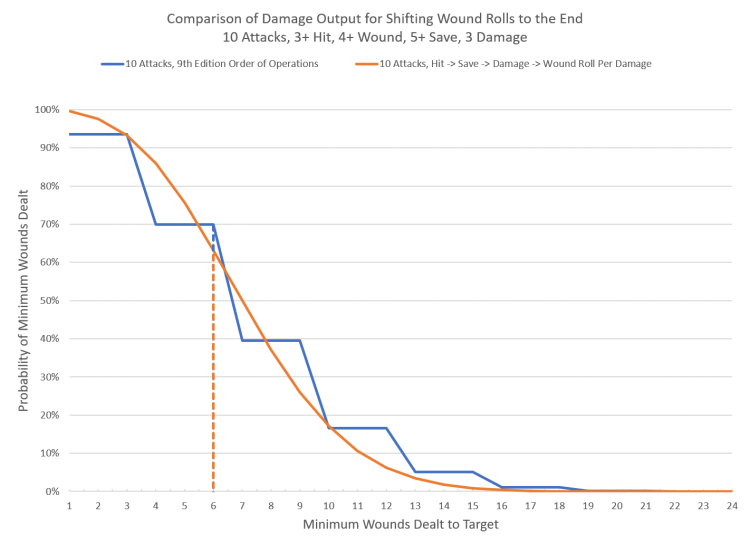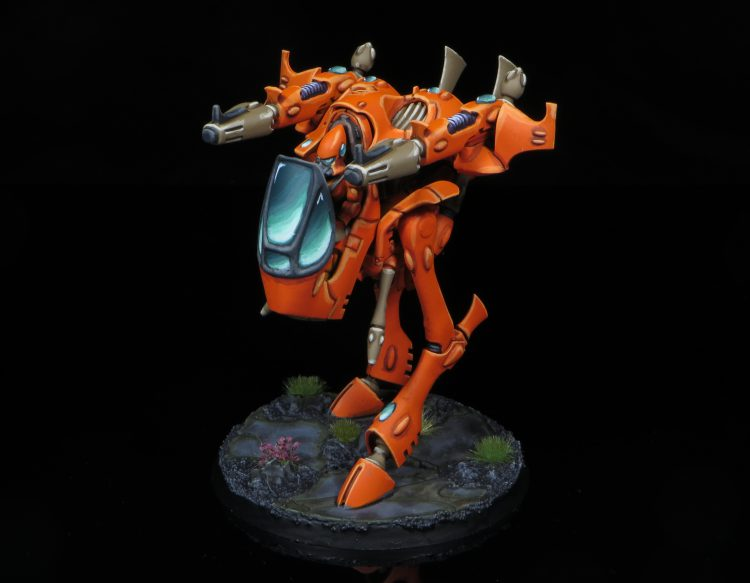War Walker. Credit: Rockfish

### Wrapping Up

Thanks to The Raven Bored for the question! It was a fun exercise to play around with, particularly in terms of finding a way to change outcomes in a meaningful fashion.

• So long as they are independent of each other, the commutative property means that changing the order in which the Hit, Wound, or Save roll is applied will not affect the probability of success.
• The commutative property also applies to re-rolls that proc off of a fixed value, such as re-rolling 1s.
• Changing the number of dice rolled by making one Wound roll for every point of damage dealt would result in a smoother probability distribution, similar to how Feel No Pain works.

Thanks for reading! If you have any feedback, feel free to drop a note in the comments below or email us at contact@goonhammer.com. If you have any questions that you would like answered in a future article, please submit them here.

This site uses Akismet to reduce spam. Learn how your comment data is processed.